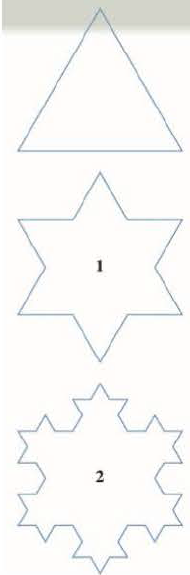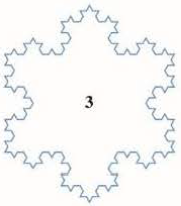Chapter 11, Problem 5P

Chapter
Section
Textbook Problem

# To construct the snowflake curve, start with an equilateral triangle with sides of length 1. Step 1 in the construction is to divide each side into three equal parts, construct an equilateral triangle on the middle part, and then delete the middle part (see the figure). Step 2 is to repeat step 1 for each side of the resulting polygon. This process is repeated at each succeeding step. The snowflake curve is the curve that results from repeating this process indefinitely. (a) Let sn, ln, and pn represent the number of sides, the length of a side, and the total length of the nth approximating curve (the curve obtained alter step n of the construction), respectively. Find formulas for sn, ln, and pn. (b) Show that pn → ∞ as n → ∞. (c) Sum an infinite series to find the area enclosed by the snowflake curve. Note: Parts (b) and (c) show that the snowflake curve is infinitely long but encloses only a finite area.FIGURE FOR PROBLEM 5(a)

To determine

To find: The formula for the number of sides sn , the length of the side ln and the total length of the nth approximating curve pn

Explanation

Calculation:

The equilateral triangle each of length, 13 of the side length of the preceding stage.

Therefore the number of sides sn , written as shown below,

s0=3s1=34s2=342s3=343

In general the number of sides sn=34n

The length of the side ln written as shown below,

l0=1l1=13l2=132l3=1

(b)

To determine

To show: The total length of the nth approximating curve. pn as n .

(c)

To determine

To find: The area enclosed by the snow flake curve.

### Still sussing out bartleby?

Check out a sample textbook solution.

See a sample solution

#### The Solution to Your Study Problems

Bartleby provides explanations to thousands of textbook problems written by our experts, many with advanced degrees!

Get Started

#### Find more solutions based on key concepts